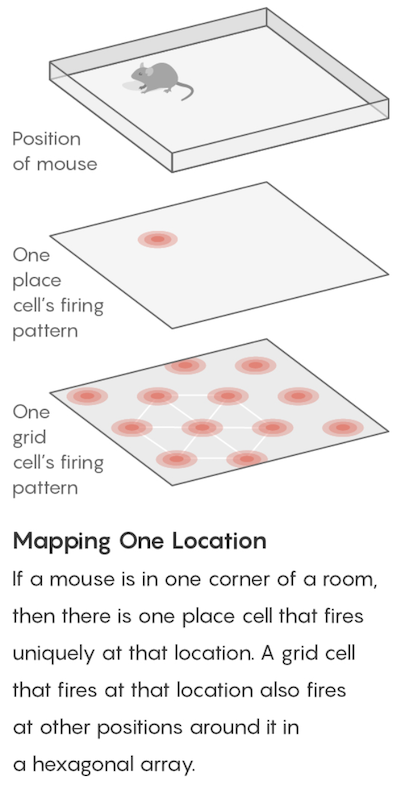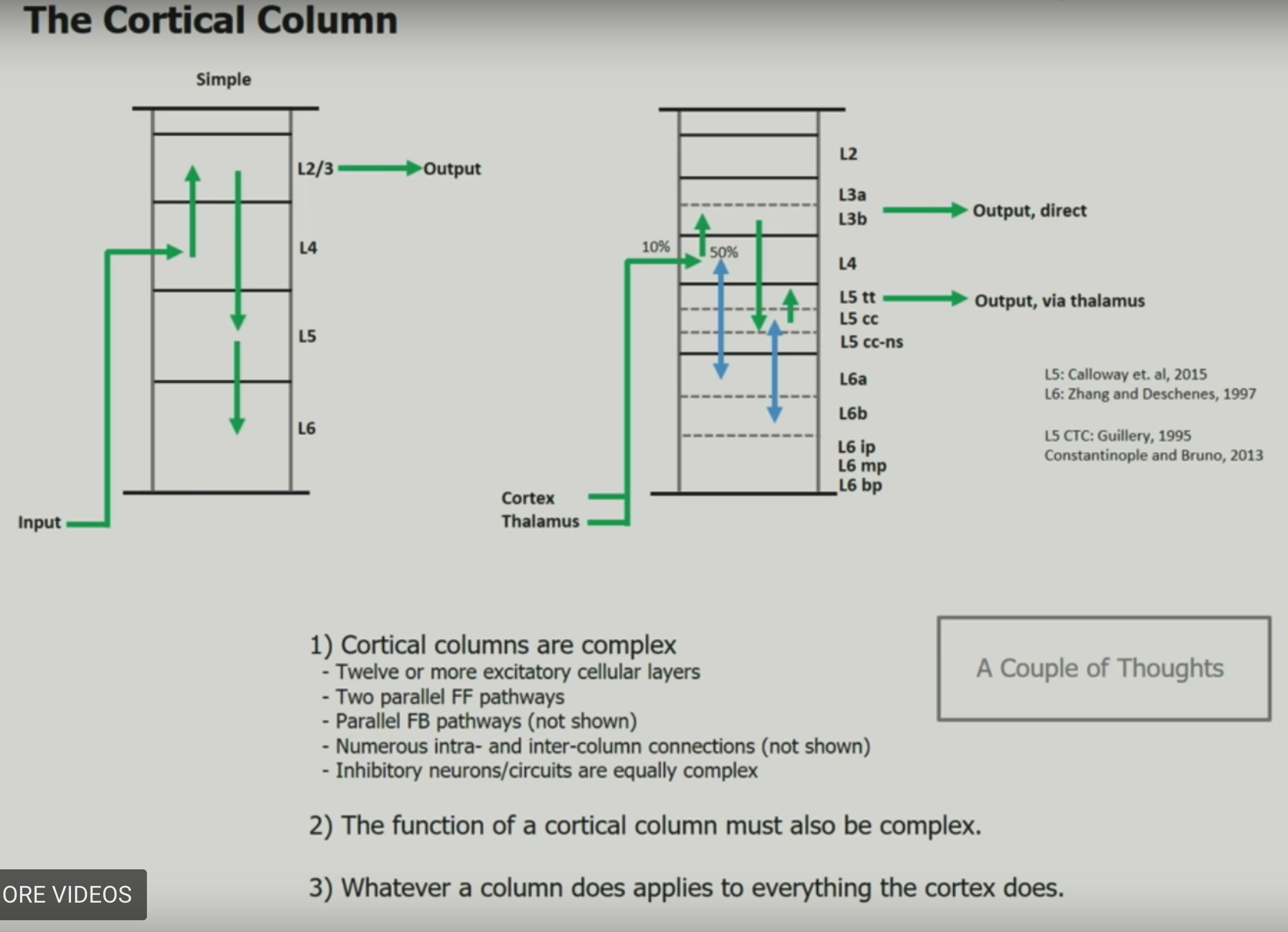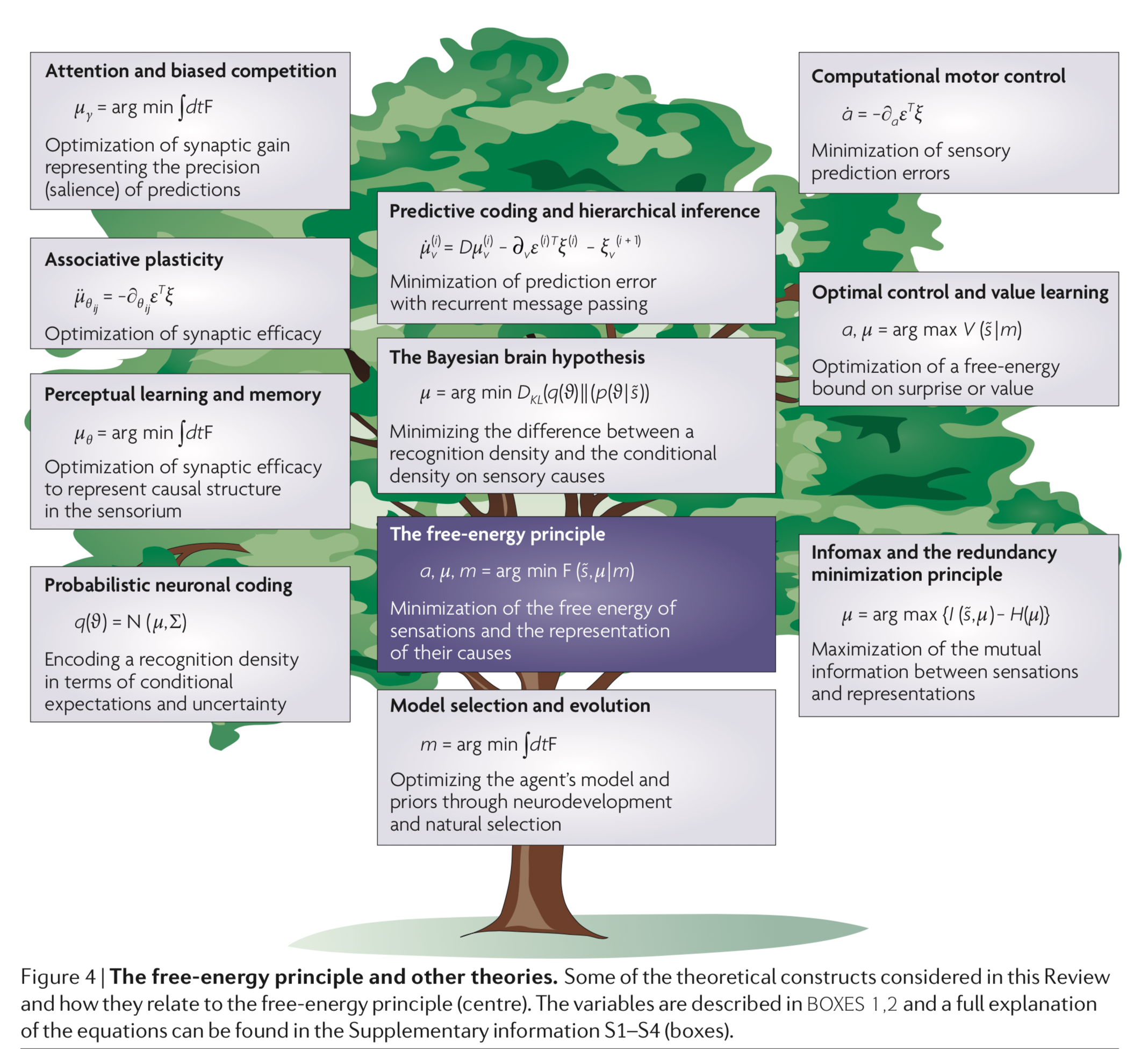comp neuro

• cognitive maps (tolman 1940s) - idea that rats in mazes learn spatial maps
• place cells (o’keefe 1971) - in the hippocampus - fire to indicate one’s current location
• remap to new locations
• grid cells (moser & moser 2005) - in the entorhinal cotex (provides inputs to the hippocampus) - not particular locations but rather hexagonal coordinate system
• grid cells fire if the mouse is in any location at the vertex (or center) of one of the hexagons
•• there are grid cells with larger/smaller hexagons, different orientations, different offsets
• can look for grid cells signature in fmri: https://www.nature.com/articles/nature08704
• other places with grid cell-like behavior
• some evidence for “time cells” like place cells for time

# high-dimensional computing

• high-level overview
• current inspiration has all come from single neurons at a time - hd computing is going past this
• the brain’s circuits are high-dimensional
• elements are stochastic not deterministic
• can learn from experience
• no 2 brains are alike yet they exhibit the same behavior
• basic question of comp neuro: what kind of computing can explain behavior produced by spike trains?
• recognizing ppl by how they look, sound, or behave
• learning from examples
• remembering things going back to childhood
• communicating with language
• HD computing overview paper
• in these high dimensions, most points are close to equidistant from one another (L1 distance), and are approximately orthogonal (dot product is 0)
• memory
• heteroassociative - can return stored X based on its address A
• autoassociative - can return stored X based on a noisy version of X (since it is a point attractor), maybe with some iteration
• this adds robustness to the memory
• this also removes the need for addresses altogether

## definitions

• what is hd computing?
• compute with random high-dim vectors
• ex. 10k vectors A, B of +1/-1 (also extends to real / complex vectors)
• 3 operations
• addition: A + B = (0, 0, 2, 0, 2,-2, 0, ….)
• multiplication: A * B = (-1, -1, -1, 1, 1, -1, 1, …) - this is XOR
• want this to be invertible, dsitribute over addition, preserve distance, and be dissimilar to the vectors being multiplied
• number of ones after multiplication is the distance between the two original vectors
• can represent a dissimilar set vector by using multiplication
• permutation: shuffles values
• ex. rotate (bit shift with wrapping around)
• multiply by rotation matrix (where each row and col contain exactly one 1)
• can think of permutation as a list of numbers 1, 2, …, n in permuted order
• many properties similar to multiplication
• random permutation randomizes
• basic operations
• weighting by a scalar
• similarity = dot product (sometimes normalized)
• A $\cdot$ A = 10k
• A $\cdot$ A = 0 (orthogonal)
• in high-dim spaces, almost all pairs of vectors are dissimilar A $\cdot$ B = 0
• goal: similar meanings should have large similarity
• normalization
• for binary vectors, just take the sign
• for non-binary vectors, scalar weight
• data structures
• these operations allow for encoding all normal data structures: sets, sequences, lists, databases
• set - can represent with a sum (since the sum is similar to all the vectors)
• can find a stored set using any element
• if we don’t store the sum, can probe with the sum and keep subtracting the vectors we find
• multiset = bag (stores set with frequency counts) - can store things with order by adding them multiple times, but hard to actually retrieve frequencies
• sequence - could have each element be an address pointing to the next element
• problem - hard to represent sequences that share a subsequence (could have pointers which skip over the subsquence)
• soln: index elements based on permuted sums
• can look up an element based on previous element or previous string of elements
• could do some kind of weighting also
• pairs - could just multiply (XOR), but then get some weird things, e.g. A * A = 0
• can use these to index (address, value) pairs and make more complex data structures
• named tuples - have smth like (name: x, date: m, age: y) and store as holistic vector $H = N*X + D * M + A * Y$
• individual attribute value can be retrieved using vector for individual key
• representation substituting is a little trickier….
• we blur what is a value and whit is a variable
• can do this for a pair or for a named tuple with new values
• this doesn’t always work
• examples
• context vectors
• standard practice (e.g. LSA): make matrix of word counts, where each row is a word, and each column is a document
• HD computing alternative: each row is a word, but each document is assigned a few ~10 columns at random
• thus, the number of columns doesn’t scale with the number of documents
• can also do this randomness for the rows (so the number of rows < the number of words)
• can still get semantic vector for a row/column by adding together the rows/columns which are activated by that row/column
• this examples still only uses bag-of-words (but can be extended to more)
• learning rules by example
• particular instance of a rule is a rule (e.g mother-son-baby $\to$ grandmother)
• as we get more examples and average them, the rule gets better
• doesn’t always work (especially when things collapse to identity rule)
• analogies from pairs
• ex. what is the dollar of mexico?

## ex. identify the language

• paper: LANGUAGE RECOGNITION USING RANDOM INDEXING (joshi et al. 2015)
• benefits - very simple and scalable - only go through data once
• equally easy to use 4-grams vs. 5-grams
• data
• train: given million bytes of text per language (in the same alphabet)
• test: new sentences for each language
• training: compute a 10k profile vector for each language and for each test sentence
• could encode each letter wih a seed vector which is 10k
• instead encode trigrams with rotate and multiply
• 1st letter vec rotated by 2 * 2nd letter vec rotated by 1 * 3rd letter vec
• ex. THE = r(r(T)) * r(H) * r(E)
• approximately orthogonal to all the letter vectors and all the other possible trigram vectors…
• profile = sum of all trigram vectors (taken sliding)
• ex. banana = ban + ana + nan + ana
• profile is like a histogram of trigrams
• testing
• compare each test sentence to profiles via dot product
• clusters similar languages - cool!
• gets 97% test acc
• can query the letter most likely to follor “TH”
• form query vector $Q = r(r(T)) * r(H)$
• query by using multiply X + Q * english-profile-vec
• find closest letter vecs to X - yields “e”

## details

• mathematical background
• randomly chosen vecs are dissimilar
• sum vector is similar to its argument vectors
• product vector and permuted vector are dissimilar to their argument vectors
• permutation distributes over both additions and multiplication
• multiplication and permutations are invertible
• comparison to DNNs
• both do statistical learning from data
• data can be noisy
• both use high-dim vecs although DNNs get bad with him dims (e.g. 100k)
• HD is founded on rich mathematical theory
• new codewords are made from existing ones
• HD memory is a separate func
• HD algos are transparent, incremental (on-line), scalable
• somewhat closer to the brain…cerebellum anatomy seems to be match HD
• HD: holistic (distributed repr.) is robust
• different names
• Tony plate: holographic reduced representation
• gayler & levi: vector-symbolic arch
• gallant & okaywe: matrix binding with additive termps
• fourier holographic reduced reprsentations (FHRR; Plate)
• …many more names
• theory of sequence indexing and working memory in RNNs
• trying to make key-value pairs
• VSA as a structured approach for understanding neural networks
• reservoir computing = state-dependent network = echos-state network = liquid state machine - try to represen sequential temporal data - builds representations on the fly

# dnns with memory

• Neural Statistician (Edwards & Storkey, 2016) summarises a dataset by averaging over their embeddings
• kanerva machine
• like a VAE where the prior is derived from an adaptive memory store

# dynamic routing between capsules

• hinton 1981 - reference frames requires structured representations
• mapping units vote for different orientations, sizes, positions based on basic units
• mapping units gate the activity from other types of units - weight is dependent on if mapping is activated
• top-down activations give info back to mapping units
• this is a hopfield net with three-way connections (between input units, output units, mapping units)
• reference frame is a key part of how we see - need to vote for transformations
• olshausen, anderson, & van essen 1993 - dynamic routing circuits
• ran simulations of such things (hinton said it was hard to get simulations to work)
• we learn things in object-based reference frames
• inputs -> outputs has weight matrix gated by control
• zeiler & fergus 2013 - visualizing things at intermediate layers - deconv (by dynamic routing)
• save indexes of max pooling (these would be the control neurons)
• when you do deconv, assign max value to these indexes
• arathom 02 - map-seeking circuits
• tenenbaum & freeman 2000 - bilinear models
• trying to separate content + style
• hinton et al 2011 - transforming autoencoders - trained neural net to learn to shift imge
• sabour et al 2017 - dynamic routing between capsules
• units output a vector (represents info about reference frame)
• matrix transforms reference frames between units
• recurrent control units settle on some transformation to identify reference frame
• notes from this blog post
• problems with cnns
• pooling loses info
• don’t account for spatial relations between image parts
• can’t transfer info to new viewpoints
• capsule - vector specifying the features of an object (e.g. position, size, orientation, hue texture) and its likelihood
• ex. an “eye” capsule could specify the probability it exists, its position, and its size
• magnitude (i.e. length) of vector represents probability it exists (e.g. there is an eye)
• direction of vector represents the instatntiation parameters (e.g. position, size)
• hierarchy
• capsules in later layers are functions of the capsules in lower layers, and since capsule has extra properties can ask questions like “are both eyes similarly sized?”
• equivariance = we can ensure our net is invariant to viewpoints by checking for all similar rotations/transformations in the same amount/direction
• active capsules at one level make predictions for the instantiation parameters of higher-level capsules
• when multiple predictions agree, a higher-level capsule is activated
• steps in a capsule (e.g. one that recognizes faces)
• receives an input vector (e.g. representing eye)
• apply affine transformation - encodes spatial relationships (e.g. between eye and where the face should be)
• applying weighted sum by the C weights, learned by the routing algorithm
• these weights are learned to group similar outputs to make higher-level capsules
• vectors are squashed so their magnitudes are between 0 and 1
• outputs a vector

# hierarchical temporal memory (htm)

• binary synapses and learns by modeling the growth of new synapses and the decay of unused synapses
• separate aspects of brains and neurons that are essential for intelligence from those that depend on brain implementation

## necortical structure

• evolution leads to physical/logical hierarchy of brain regions
• neocortex is like a flat sheet
• neocortex regions are similar and do similar computation
• Mountcastle 1978: vision regions are vision becase they receive visual input
• number of regions / connectivity seems to be genetic
• before necortex, brain regions were homogenous: spinal cord, brain stem, basal ganglia, …
•## principles

• common algorithims accross neocortex
• hierarchy
• sparse distributed representations (SDR) - vectors with thousands of bits, mostly 0s
• bits of representation encode semantic properties
• inputs
• data from the sense
• copy of the motor commands
• “sensory-motor” integration - perception is stable while the eyes move
• patterns are constantly changing
• necortex tries to control old brain regions which control muscles
• learning: region accepts stream of sensory data + motor commands
• learns of changes in inputs
• ouputs motor commands
• only knows how its output changes its input
• must learn how to control behavior via associative linking
• sensory encoders - takes input and turnes it into an SDR
• engineered systems can use non-human senses
• behavior needs to be incorporated fully
• temporal memory - is a memory of sequences
• everything the neocortex does is based on memory and recall of sequences of patterns
• on-line learning
• prediction is compared to what actually happens and forms the basis of learning
• minimize the error of predictions

## papers

• “A Theory of How Columns in the Neocortex Enable Learning the Structure of the World”
• network model that learns the structure of objects through movement
• object recognition
• over time individual columns integrate changing inputs to recognize complete objects
• through existing lateral connections
• within each column, neocortex is calculating a location representation
• locations relative to each other = allocentric
• much more motion involved
• multiple columns - integrate spatial inputs - make things fast
• single column - integrate touches over time - represent objects properly
• “Why Neurons Have Thousands of Synapses, A Theory of Sequence Memory in Neocortex”
• learning and recalling sequences of patterns
• neuron with lots of synapses can learn transitions of patterns
• network of these can form robust memory

# forgetting

• Continual Lifelong Learning with Neural Networks: A Review
• main issues is catastrophic forgetting / stability-plasticity dilemma
•• 2 types of plasticity
• Hebbian plasticity (Hebb 1949) for positive feedback instability
• compensatory homeostatic plasticity which stabilizes neural activity
• approaches: regularization, dynamic architectures (e.g. add more nodes after each task), memory replay

# deeptune-style

• ponce_19_evolving_stimuli: https://www.cell.com/action/showPdf?pii=S0092-8674%2819%2930391-5
• bashivan_18_ann_synthesis
• use kernel regression from CNN embedding to calculate distances between preset images
• select preset images
• verified with macaque v4 recording
• currently only study that optimizes firing rates of multiple neurons
• pick next stimulus in closed-loop (“adaptive sampling” = “optimal experimental design”)
• J. Benda, T. Gollisch, C. K. Machens, and A. V. Herz, “From response to stimulus: adaptive sampling in sensory physiology”
• find the smallest number of stimuli needed to fit parameters of a model that predicts the recorded neuron’s activity from the stimulus

• maximizing firing rates via genetic algorithms

• maximizing firing rate via gradient ascent

• C. DiMattina and K. Zhang,“Adaptive stimulus optimization for sensory systems neuroscience”](https://www.frontiersin.org/articles/10.3389/fncir.2013.00101/full)

• 2 general approaches: gradient-based approaches + genetic algorithms
• can put constraints on stimulus space
• might want iso-response surfaces
• maximally informative stimulus ensembles (Machens, 2002)
• model-fitting: pick to maximize info-gain w/ model params
• using fixed stimulus sets like white noise may be deeply problematic for efforts to identify non-linear hierarchical network models due to continuous parameter confounding (DiMattina and Zhang, 2010)
• use for model selection

# population coding

• saxena_19_pop_cunningham: “Towards the neural population doctrine”
• correlated trial-to-trial variability
• Ni et al. showed that the correlated variability in V4 neurons during attention and learning — processes that have inherently different timescales — robustly decreases
• ‘choice’ decoder built on neural activity in the first PC performs as well as one built on the full dataset, suggesting that the relationship of neural variability to behavior lies in a relatively small subspace of the state space.
• decoding
• more neurons only helps if neuron doesn’t lie in span of previous neurons
• encoding
• can train dnn goal-driven or train dnn on the neural responses directly
• testing
• important to be able to test population structure directly
• population vector coding - ex. neurons coded for direction sum to get final direction
• reduces uncertainty
• correlation coding - correlations betweeen spikes carries extra info
• independent-spike coding - each spike is independent of other spikes within the spike train
• position coding - want to represent a position
• for grid cells, very efficient
• sparse coding
• hard when noise between neurons is correlated
• measures of information
• eda
• plot neuron responses
• calc neuron covariances

# interesting misc papers

• berardino 17 eigendistortions
• Fisher info matrix under certain assumptions = $Jacob^TJacob$ (pixels x pixels) where Jacob is the Jacobian matrix for the function f action on the pixels x
• most and least noticeable distortion directions corresponding to the eigenvectors of the Fisher info matrix
• gao_19_v1_repr
• don’t learn from images - v1 repr should come from motion like it does in the real world
• repr
• vector of local content
• matrix of local displacement
• why is this repr nice?
• separate reps of static image content and change due to motion
• disentangled rotations
• learning
• predict next image given current image + displacement field
• predict next image vector given current frame vectors + displacement
• kietzmann_18_dnn_in_neuro_rvw
• friston_10_free_energy
•